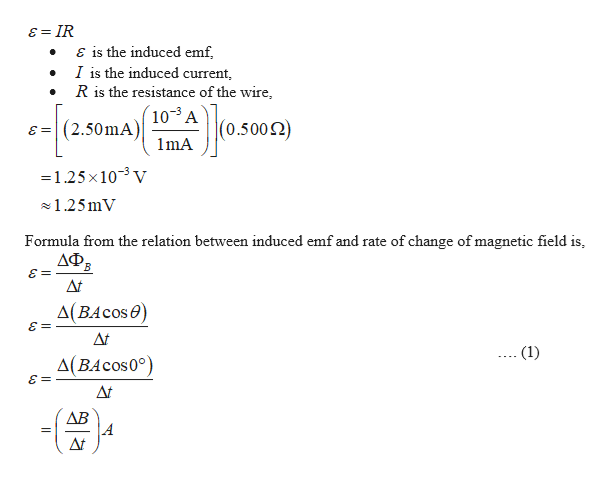Question
5 views

A circular loop of wire of resistance R = 0.500 Ω and radius
r = 8.00 cm is in a uniform magnetic field directed out of the
page as in Figure P20.54. If a clockwise current of I = 2.50
mA is induced in the loop, (a) is the magnetic field increasing
or decreasing in time? (b) Find the rate at which the field is
changing with time.

check_circle

Step 1

The clockwise induced current in the loop produces a flux which is directed into the page through the area enclosed by the loop.

Since the flux generated in the circular loop opposes the change in flux due to the external magnetic field, the outward directed flux due to the external filed must be increasing in magnitude. Hence, the magnitude of the external field itself must be increasing in time.

Step 2

b).

Formula to calculate the emf induc...help_outlineImage Transcriptioncloseɛ = IR E is the induced emf, I is the induced current, R is the resistance of the wire, (10-³ A Josmay (2.50mA) =3 1mA =1.25x10-3 V 1.25mV Formula from the relation between induced emf and rate of change of magnetic field is, ДФЕ. = 3 At A(BAcos0) At (1) Д(ВАсos0°) At ДВ At fullscreen

### Want to see the full answer?

See Solution

#### Want to see this answer and more?

Solutions are written by subject experts who are available 24/7. Questions are typically answered within 1 hour.*

See Solution
*Response times may vary by subject and question.
Tagged in
SciencePhysics

### Alternating Current